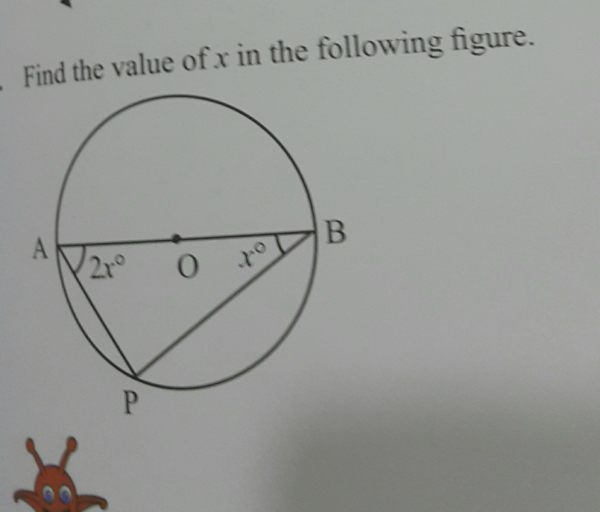">

# Find the value of x in the following figure:The angle APB is in a semicircle. So its value is 90 degree

Sum of angles of the triangle APB = x + 2x + 90 = 180

3x + 90 = 180

3x = 180 - 90 = 90

or x = 90/3 = 30 degrees  Answer

Updated on: 10-Oct-2022

28 Views